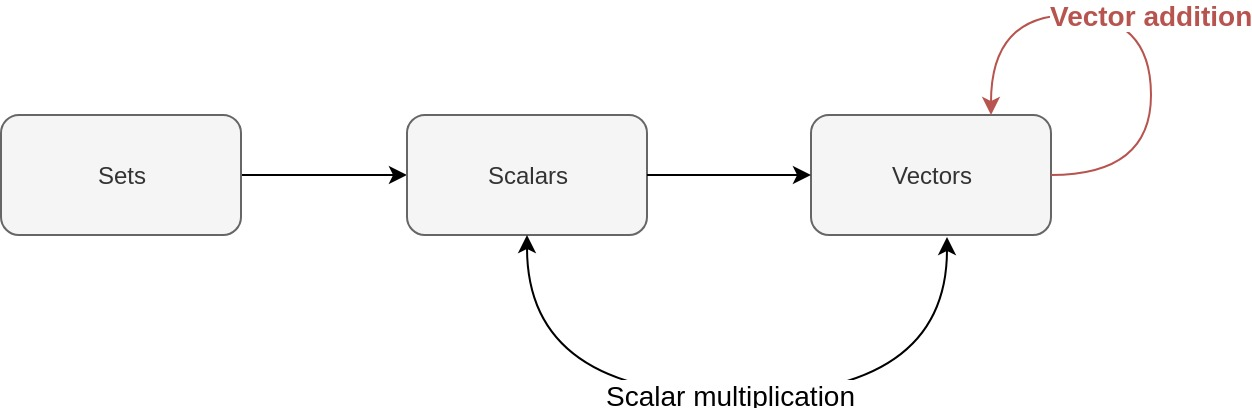# Deep (learning) like Jacques Cousteau - Part 5 - Vector addition

4 minute read

(TL;DR: You can add vectors that have similar dimensions.)

LaTeX and MathJax warning for those viewing my feed: please view directly on website!You want to know how to rhyme, you better learn how to add
It’s mathematics

Mos Def in ‘Mathematics’

Last time, we learnt about scalar multiplication. Let’s get to adding vectors together!# Today’s topic: vector addition

We will follow the notation in Goodfellow, Ian, et al.. If our vector $\boldsymbol{x}$ has $n$ elements that are real numbers, then we can say that $\boldsymbol{x}$ is a $\boldsymbol{n}$-dimensional vector.

We can also say that $\boldsymbol{x}$ lies in some set of all vectors that have the same dimensions as itself. This might be a bit abstract at first, but it’s not too bad at all.

Let’s define two vectors:

They have two elements. They are, therefore, two-dimensional vectors.

What are some other two dimensional vectors made up of real numbers? We could have:

There are infinitely many vectors with two elements that we could come up with! How can we describe this infinite set of vectors made up of real numbers? We can say that our two-dimensional vectors made up of real numbers lie in this set:

What in the world does this mean?

## Cartesian products

To understand what the above product means, let’s use a simplified example. Let’s define sets of integers like so:

What is the result of $X \times Y$? We can depict this operation in a matrix:

What we’re doing here is taking an arbitrary element from our first set, $X$. We are then pairing it up with a second arbitrary element from the set, $Y$. The entries in the matrix form a set of ordered pairs (i.e. the order of elements in the pair of numbers matter) which can be defined like so:

This is called the Cartesian product of two sets. Translating the equation into plain English gives us this:

Now let’s replace the terminology of ordered pairs with the word vectors. If we depict the vectors as transposed row vectors, our matrix now looks like this:

Now let’s replace sets $X$ and $Y$ with the set of real numbers, $\mathbb{R}$. The Cartesian product then becomes:

We now have a set of ordered pairs which are drawn from the Cartesian product of the set of real numbers with itself! If we again replace the term ‘ordered pairs’ with ‘vectors’, we now have the set of all two dimensional, real number vectors. We can finally say this about the vectors we defined earlier:

Hooray!

Now it’s not difficult to see that $\mathbb{R}^n$ is the set formed by taking the $n$-ary Cartesian product of the set of real numbers. We can now quite easily generalise to vectors of $n$ dimensions.

## Get to the point! When can we add vectors?

If we have vectors of equal dimensions, we simply add the elements of the vectors, one element at a time! The resulting vector is a vector of the same dimensions as the vectors that were just added.

Let’s use our example vectors from before:

We know that $x, y \in \mathbb{R}^2$. We now know that $x + y \in \mathbb{R}^2$. Let’s add them:

Easy!

# How can we add vectors in R?

Some operations on vectors are defined differently to those that are defined in linear algebra.

When we have two numeric vectors with the same number of elements, we can add them just like we saw above:

x <- c(1, 2, 3)
y <- c(2, 3, 4)

x +  y

##  3 5 7


But what if we try to add two numeric vectors with different numbers of elements?

x <- c(1, 2)
y <- c(1, 1, 1, 1)
x + y

##  2 3 2 3


What the hell just happened? This isn’t what we have just learnt! How is it possible to add these two vectors given the mathematical definition of vector addition?

If we look at the help page for +, we can find our answer:

?+


The binary operators return vectors containing the result of the element by element operations. If involving a zero-length vector the result has length zero. Otherwise, the elements of shorter vectors are recycled as necessary (with a warning when they are recycled only fractionally).

This is what R is doing:

• For the first two elements of the longer vector, R is adding the shorter vector to it in the above mathematically defined way.
• When it runs out of elements in the shorter vector, R goes back to the first element of the shorter vector and adds this to the next element of the longer vector.
• R continues like this until it runs out of elements in the longer vector.

(Man, that’s a lot of use of the word vector!)

In this way, R allows you to add vectors of unequal length. We also told that some other operators also behave in this way:

The operators are + for addition, - for subtraction, * for multiplication, / for division and ^ for exponentiation.

These aren’t our focus here so let’s move on.

# What about adding scalars to vectors?

In our standard mathematical definition, we can’t add scalars to vectors. But we can in R!

We simply add the scalar to our vector, one element at a time:

x <- 1
y <- c(1, 2, 3)

x + y

##  2 3 4


# Conclusion

We learnt how to add vectors. We also learnt a little bit more about sets. We learnt that R can behave differently to our mathematical definitions of vector addition. Let’s now move onto dot products!

Categories:

Updated: